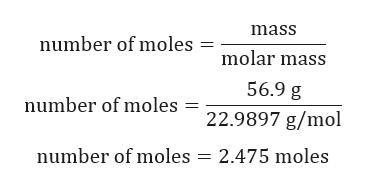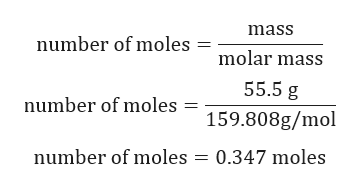Question
check_circleExpert Solution
Step 1

Calculation for number of moles of Na:help_outlineImage Transcriptionclosemass number of moles molar mass 56.9 g number of moles 22.9897 g/mol number of moles 2.475 moles fullscreen
Step 2

Calculation for number of moles of Br2:help_outlineImage Transcriptionclosemass number of moles molar mass 55.5 g number of moles = 159.808g/mol number of moles = 0.347 moles fullscreen
Step 3

Identifying limiting reactant:

From the above chemical reaction, it is evident that 2 moles of Na react with 1 mole of Br2.

Number of moles of Na = 2.475 moles/2 = 1....

### Want to see the full answer?

See Solution

#### Want to see this answer and more?

Solutions are written by subject experts who are available 24/7. Questions are typically answered within 1 hour*

See Solution
*Response times may vary by subject and question
Tagged in

### Chemistry